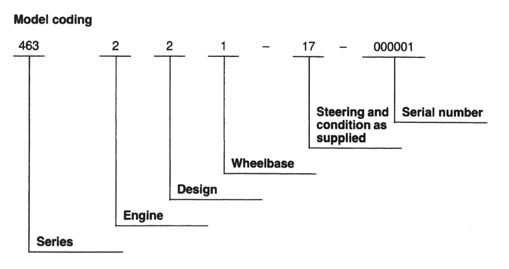Model coding

Mercedes-Benz Gelaendewagen 460 (1979 - 1990)
and 461/463 G-Class series (1990 - 2011) serial numbers (VIN#)

So, you were lucky enough to get your hands on one of the best 4x4 around and want to find out more about your vehicle.
Here is what the VIN numbers mean
(list is not complete yet):

## Gelaendewagen site production line up workshop manuals owners manuals Webasto manuals which wheels will fit? new 463 features intro photo & poster CD Front wheel bearing tip Drive shaft grease nipple tip 35 inch tires on a G links to other G sites G-Class history Images of my Gs www.4xw4abc.com Rubicon-Trail.comVIN decoding and data card for your specific vehicle Series 460 461 463 . Engine 2 = gasoline 3 = Diesel 2 = gasoline 3 = Diesel 4 = military ? 2 = gasoline 3 = Diesel . Design 1 = convertible 2 = van, chassis w cab 3 = station wagon 4 = pickup 9 = armored 1 = ? 2 = van, chassis with cab 3 = station wagon 4 = pickup 5 = open vehicle 6 = open vehicle 0 = open vehicle 0 = convertible 2 = station wagon 3 = station wagon 4 = station wagon 5 = convertible 7 = AMG Kompressor . Wheelbase 0 (BA1) = convertible 2400 0 (BA3) = station, 2400 0 (BA4) = van, 2850 0 (BA7) =open vehicle, 2400 1 (BA4) = van, 2850 2 (BA1) = convertible 2400 2 (BA3) =station, 2400 2 (BA7) =open vehicle, 2400 2 (BA9) =chassis w cab, 3120 3 (BA4) = van, 2850 3 (BA5) = pickup, 2850 3 (BA6) = station, 2850 3 (BA10) = open vehicle, 3120 4 (BA9) =chassis w cab, 3120 5 (BA9) =chassis w cab, 3120 6 (BA1) = convertible, Italy 6 (BA3) =station, 2400, Italy 7 (BA1) = convertible 7 (BA3) =station, 2400 7 (BA6) = station, 2850 7 (BA9) =chassis w cab, 3120 8 (BA1) = convertible 2400 8 (BA3) = station, 2400 8 (BA4) = van, 2850 8 (BA6) = station, 2850 8 (BA7) =open vehicle, 2400 9 (BA3) = station, 2400 9 (BA5) = pickup, 2850 9 (BA6) = station, 2850 9 (BA9) =chassis w cab, 3120 9 (BA10) = open vehicle, 3120 0 (BA3) = station, 2400 0 (BA7) =open vehicle, 2400 1 (BA5) = pickup 2850 1 (BA6) = station, 2850 1 (BA7) =open vehicle, 2400 1 (BA10) = open vehicle, 3120 1 (BA11) = double cab, 3120 or 3400 2 (BA4) = van, 2850 2 (BA6) = station, 2850 2 (BA9) =chassis w cab, 3120 2 (BA10) = open vehicle, 3120 3 (BA4) = van, 2850 3 (BA9) = chassis w cab 3400 3 (BA10) = open vehicle, 3120 4 (BA4) = van, 2850 4 (BA9) =chassis w cab, 3120 4 (BA10) = open vehicle, 3120 5 (BA3) = station, 2400 5 (BA7) =open vehicle, 2400 5 (BA9) =chassis w cab, 3120 5 (BA10) = open vehicle, 3120 6 (BA4) = van, 2850 6 (BA6) = station, 2850 6 (BA7) = open vehicle, 2400 6 (BA10) = open vehicle, 3120 7 (BA3) =station, 2400 7 (BA7) =open vehicle, 2400 7 (BA9) =chassis w cab, 3120 7 (BA10) =open vehicle, 2850 8 (BA3) = station, 2400 8 (BA4) = van, 2850 8 (BA6) = station, 2850 8 (BA9) =chassis w cab, 3120 8 (BA10) = open vehicle, 3120 9 (BA4) = van, 2850 9 (BA5) = pickup, 2850 9 (BA6) = station, 2850 9 (BA9) =chassis w cab, 3120 0 (BA1) = convertible, 2400 0 (BA3) = station, 2400 0 (BA6) = station, 2850 1 (BA6) = station, 2850 2 (BA3) =station, 2400 3 (BA6) = station, 2850 4 (BA1) = convertible, 2400 4 (BA3) = station, 2400, 5 (BA6) = station, 2850 6 (BA1) = convertible 2400 6 (BA6) = station, 2850 7 (BA1) = convertible, 2400 7 (BA3) = station, 2400 8 (BA1) = convertible, 2400 8 (BA3) = station, 2400 8 (BA6) = station, 2850 9 (BA1) = convertible 2400 9 (BA6) = station, 2850 . Steering and condition as supplied 17 = LH 27 = RH 13 = LH 1X = LH 23 = RH 2X = RH 50 = vehicle set 52 = LH, chassis with cab 53 = LH CKD, complete vehicle 63 = RH CKD, complete vehicle 13 = LH 1X = LH 23 = RH 2X = RH 19 = LH AMG 50 = vehicle set 52 = LH, chassis with cab 53 = LH CKD, complete vehicle 63 = RH CKD, complete vehicle MBUSA VIN number coding for vehicles starting in 2002 first 3 digits WDC = DaimlerChrysler AG manufacturer fourth digit Y = 463 chassis designation fifth digit R = gas engine type 6th + 7th digit 49 49 = G500 46 = G55 body style eighth digit E = 6001 - 7000 lbs. gross vehicle weight ninth digit 5 for factory use test number tenth digit 2 2 = 2002, 3 = 2003, 4 = 2004 model year eleventh digit X = Graz, Austria manufacturing plant last six digits 126776 serial number VIN If you have an answer for the lines where I have put a question mark - please let me know. contact me © 1998-2012 Harald Pietschmann